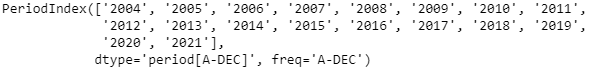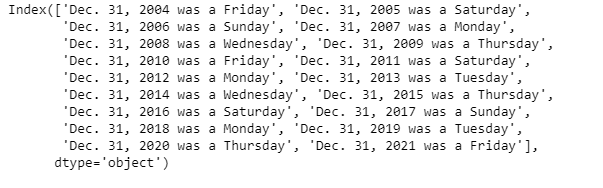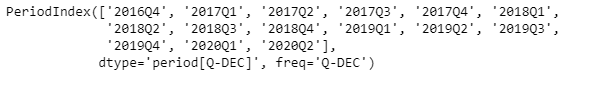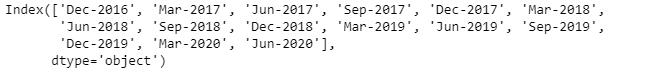Skip to content
Related Articles
Python | Pandas PeriodIndex.strftime
• Last Updated : 06 Jan, 2019

Python is a great language for doing data analysis, primarily because of the fantastic ecosystem of data-centric python packages. Pandas is one of those packages and makes importing and analyzing data much easier.

Pandas` PeriodIndex.strftime()` function return an array of formatted strings specified by date_format, which supports the same string format as the python standard library.

Syntax : PeriodIndex.strftime(date_format)

Parameters :
date_format : date format string (e.g. “%Y-%m-%d”)

Return : ndarray of formatted strings

Example #1: Use `PeriodIndex.strftime()` function to print the given PeriodIndex object in the specified date_format.

 `# importing pandas as pd``import` `pandas as pd`` ` `# Create the PeriodIndex object``pidx ``=` `pd.PeriodIndex(start ``=` `'2004-11-11 02:45:21 '``,``               ``end ``=` `'2021-5-21 8:45:29'``, freq ``=` `'Y'``)`` ` `# Print the PeriodIndex object``print``(pidx)`

Output :Now we will use the `PeriodIndex.strftime()` function to return the each period element in (‘%b. %d, %Y was a %A’) format.

 `# return the PeriodIndex in specified format``pidx.strftime(``'% b. % d, % Y was a % A'``)`

]

Output :As we can see in the output, the `PeriodIndex.strftime()` function has returned an Index object containing each element of the given PeriodIndex object in the specified format.

Example #2: Use `PeriodIndex.strftime()` function to print the given PeriodIndex object in the specified date_format.

 `# importing pandas as pd``import` `pandas as pd`` ` `# Create the PeriodIndex object``pidx ``=` `pd.PeriodIndex(start ``=` `'2016-10-12 11:12:02'``, ``            ``end ``=` `'2020-04-12 11:32:12'``, freq ``=` `'Q'``)`` ` `# Print the PeriodIndex object``print``(pidx)`

Output :Now we will use the `PeriodIndex.strftime()` function to return the each period element in (‘%b-%Y’) format.

 `# return the PeriodIndex in specified format``pidx.strftime(``'% b-% Y'``)`

]

Output :As we can see in the output, the `PeriodIndex.strftime()` function has returned an Index object containing each element of the given PeriodIndex object in the specified format.

Attention geek! Strengthen your foundations with the Python Programming Foundation Course and learn the basics.

To begin with, your interview preparations Enhance your Data Structures concepts with the Python DS Course. And to begin with your Machine Learning Journey, join the Machine Learning – Basic Level Course

My Personal Notes arrow_drop_up